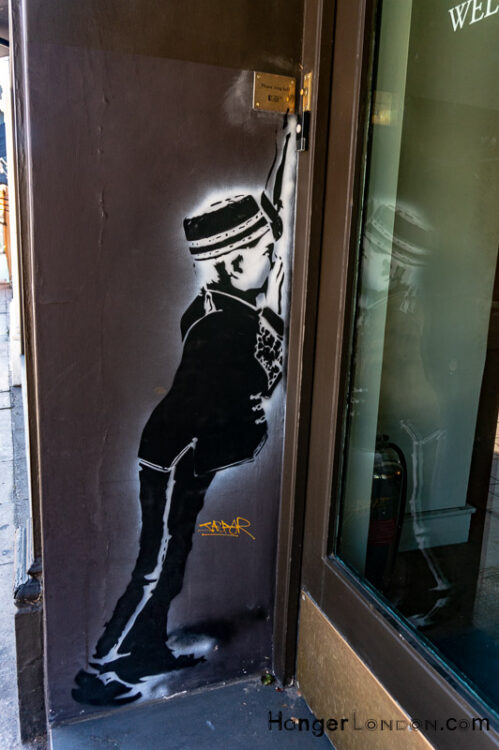# 22nd October 1963 TV reveals the London East End Housing shortage

22nd October 1963 TV series World in Action shows the East End Slums

Article Rating
Subscribe
Notify of
Inline Feedbacks

## Maths Quiz: Test your basic Maths

/9
10
Created on

Mathematic Quiz - Prime and Composite Numbers

Math quiz helps us to increase our knowledge

1 / 9

is 601 PRIME number or a COMPOSITE number?

2 / 9

is 29 PRIME number or a COMPOSITE number?

3 / 9

is 987 PRIME number or a COMPOSITE number?

4 / 9

is 908 PRIME number or a COMPOSITE number?

5 / 9

is 61 a Prime number or a Composite number?

6 / 9

is 529 PRIME number or a COMPOSITE number?

7 / 9

is 16 PRIME number or a COMPOSITE number?

8 / 9

is 850 PRIME number or a COMPOSITE number?

9 / 9

is 441 PRIME number or a COMPOSITE number?